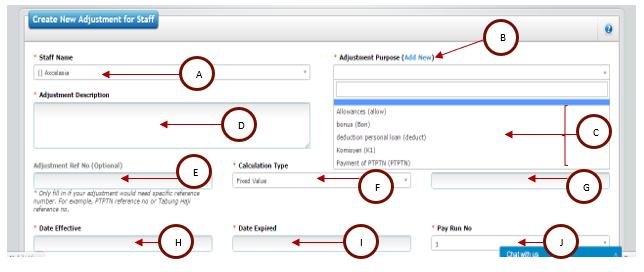1. Next, you can add allowance or deduction for your staff. Click on “ADD NEW” button to select and key in allowance or deduction for your staff.2. You will reach the page which requires you to key in the information regarding the allowance of deduction. Fill in the required information. Refer the picture below.Description:

 A Select staff which you wish to add allowance or deduction. B Please click on “Add New” if there is no suitable option for allowance or deduction type. C Select allowance or deduction type. D Fill in adjustmen description the allowance/deduction. E Fill in reference number if available. Usually, reference number is used (as account number) if deduction is related to PTPTN, Tabung Haji, etc. This reference number will be shown in the allowance/salary deduction report. F Select calculation type for this allowance.Select “Fixed Value” if allowance/deduction is fixed. For example, the allowance/deduction is always RM100. Therefore, select “Fixed Value” and enter number 100 in box number 7.Select “Based on Salary Calculation Formula” if allowance/deduction calculation is according to salary calculation. For example, if salary calculation contains deduction for unpaid leave, this allowance will also be deducted according to the same formula based on the number of unpaid leave.Select “Percentage of Basic Salary” if the allowance/deduction is calculate based on percentage of basic salary. For instance, if the percentage is 10% and the staff basic salary is RM2000, the allowance/deduction will automatically be calculated as RM200.Select “Percentage of gross salary” if the allowance/deduction is calculate based on gross salary. For instance, if the percentage if 10% and staff gross salary for the month after taking into account unpaid leave is RM1850, therefore the allowance/deduction will automatically be calculated as RM185. G Key in the amount in number, based on your selection from #F:“Fixed Value”: Key in the allowance/deduction amount. System will use this amount without any changes for calculation during salary processing.“Based on Salary Calculation Formula”: Key in the allowance/deduction amount. System will use the same formula as salary calculation to calculate total allowance or deduction.“Percentage of Basic Salary”: Key in total amount in the form of percentage from basic salary. If it is 10%, key in 10 in the box.“Percentage of Gross Salary”: Key in the total amount in the form of percentage of gross salary. If it is 10%, key in 10 in the box. H Enter the effective date of the allowance/deduction. System will calculate this allowance/deduction automatically during salary processing based on this effective date. I Enter expiry date of the allowance/deduction. System will not calculate the allowance/deduction after the expiry date automatically. Therefore, you do not have to deactivate this allowance when completed. J Select “Pay run no” of the allowance. If salary payment is made once a month, please select no.1. If salary payment is made twice a month, please select no. 2.